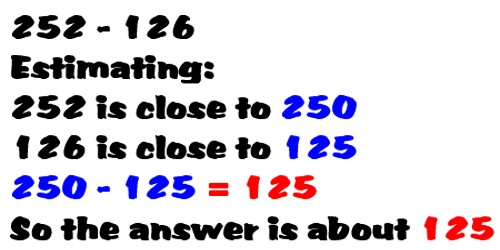Subtraction Using Estimation

A quick way to estimate the difference between two numbers is to round each number and then subtract the rounded numbers. This probably won’t be the exact answer but it may be close enough for some purposes.

How to Estimate a difference by rounding?

• Round each term that will be subtracted
• Subtract the rounded numbers

An estimate can sometimes be improved. If the sum of 645 – 450 were estimated, we would round 645 to 600 and 450 to 500. The estimate would be 600 – 500 or 100. One number was rounded down and the other was rounded up. The number 645 was rounded down by 45 and 450 was rounded up by 50. Adding 45 + 50 gives 95, which rounds to 100. Therefore, a better estimate would be 200. The actual difference is 195.

How to Improve the Estimate?

• Round each term that will be subtracted
• Subtract the rounded numbers
• If one is rounded down and the other up see if the amount of rounding is more than 50. If it is add 100 to or subtract 100 from the estimate.
• If both numbers are rounded down or both are rounded up a closer estimate will not be produced by this method.Example:

Problem: “Julian adds 34 567 and 478 on his calculator and gets 34 089.” Write 34 567 + 478 = 34 089 on the board. Discuss why Julian must be wrong.